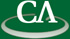## Algorithms for factoring square-free polynomials over finite fields.

### Chelsea Richards, CECM.

Thursday August 6th, in K9509 at 10:30am.

```Given a polynomial in GF(q)[x], there are simple well known algorithms for
determining its square free part.  Assuming a(x) is a monic square-free polynomial
of degree n, we will present four algorithms from the literature for determining
its complete factorization over GF(q).
```